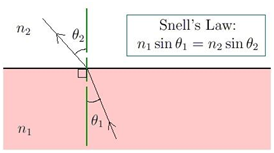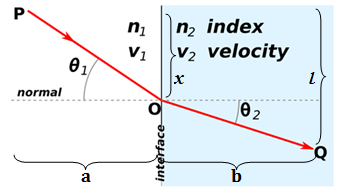# Snells Law Formula

Snell’s law tells us the degree of refraction and relation between the angle of incidence, the angle of refraction and refractive indices of given pair of media. We know that light experiences the refraction or bending when it travels from one medium to another medium. Snell’s law predicts the degree of the bend. It is also known as the law of refraction. In 1621, Willebrord Snell discovered the law of refraction, hence called Snell’s law.

Snell’s law is defined as “The ratio of the sine of the angle of incidence to the sine of the angle of refraction is a constant, for the light of a given colour and for the given pair of media”. Snell’s law formula is expressed as:

##### $\frac{sin\;i}{sin\;r}=constant=\mu$

Where i is the angle of incidence and r is the angle of refraction. This constant value is called the refractive index of the second medium with respect to the first.

The following is a diagrammatic representation:The normal on the surface is used to gauge the angles that the refracted ray creates at the contact point. n1 and n2 are the two different mediums that will impact the refraction.

Derivation:

Let’s derive Snell’s law formula using Fermat’s principle. Fermat’s principle states that “light travels in the shortest path that takes the least time”.Let the refractive index of medium 1 and medium 2 are n1 and n2  respectively. Light enters from medium 1 to medium 2 through the point O. Here, θ1 is the angle of incidence; θ2 is the angle of refraction.

The traveling velocities of light in medium 1 and medium 2 are given by:

##### $v_{2}=\frac{c}{n_{2}}$

Where ‘c’ is the speed of light in vacuum

Let T be the time required for the light to travel from point P to point Q.

##### $\frac{x}{\sqrt{x^{2}+a^{2}}}+ sin\theta_{1}$

Substitute the values in equation 1, we get

##### $\frac{sin\theta1 }{v_{1}}=\frac{sin\theta_{2} }{v_{2}}$

Substitute the values of v1 and v2,

##### $n_{1}sin\theta_{1}=n_{2}sin\theta_{2}$

Hence proved

Applications of Snell’s Law Formula in Real Life:

Snell’s law has a wide range of applications in the physics especially in the branch of optics. It is used in optical apparatus such as eyeglasses, contact lenses, cameras, rainbows. There is an instrument called a refractometer that uses Snell’s law to calculate the refractive index of liquids. It is used all the time in the candy-making industry.

Solved Examples:

Question 1: If a ray is refracted at an angle of 14° and the refractive index is 1.2, compute the angle of incidence.

Solution: Given,

Angle of refraction, r = 14°

Refractive index, μ = 1.2

Using Snell’s law formula,

##### $i=sin^{-1}\left ( 0.288 \right )=16.7$

Question 2: If the angle of incidence is 25° and angle of refraction is 32°, find the refractive index of the media.

Solution: Given,

Angle of incidence, i = 25°

Angle of refraction, r = 32°

Using Snell’s law formula,

##### $\mu =0.7975\cong 0.8$

To solve more problems on the topic, download Byju’s -The Learning App.